Technical Article

# Transistor Sizing in VLSI Design Using the Linear Delay Model

November 16, 2020 by Tosin Jemilehin

## In this article, we will learn how to find the optimal size of a transistor/logic gate present in a larger circuit to provide the desired performance using the linear delay model.

In this continuation of our series on transistor sizing in VLSI, we'll go over the third and final model in our series, the linear delay model. Be sure to check out the previous articles if you'd like to learn about the linear-RC delay model and the popular Elmore delay model.

The linear delay model provides a simpler approach to model the delay of large RC distributed circuits in scenarios where, as we observed at the end of the last article, the Elmore delay gets complicated or inaccurate.

In this article, we will learn how to find the optimal size of a transistor/logic gate present in a larger circuit to provide the desired performance.

### What Does the Linear Delay Do?

Recall the previous article, where we used the Elmore delay to linearize an equivalent RC circuit model into:

$t_{pd}=(p+gh)3RC$

where p = parasitic delay.

$h=\textit{fanout} =\frac {\textit{Input Capacitance}}{\textit{Output Capacitance}}$

$g=\textit{logical effort}$

With this expression, we can compute the optimal size of a multistage MOS circuit.

### What Is Logical Effort?

Logical effort is the ratio of the effective input capacitance of a gate to the input capacitance of an inverter. Effective capacitance in this sense implies the capacitance presented at the input.

Check out the inverter shown in the figure below, where PMOS is twice the unit size of NMOS to give equal rise/fall time.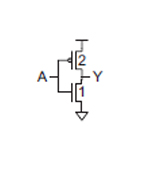##### Figure 1. Unit inverter circuit. All images adapted from CMOS VLSI Design (4th ed.)1 by Neil H.E. Weste and David Money Harris

$\textit{logical effort} (g) = \frac {\textit{effective capacitance}}{\textit{effective capacitance of an inverter}} =\frac{3C}{3C}=1$

For a 3-input NAND gate shown below, the capacitor presented at the input B is (3C + 2C = 5C).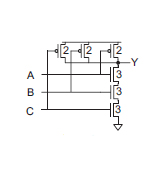##### Figure 2. 3-input NAND gate

Therefore the logical effort is

$\textit{logical effort} (g) = \frac {\textit{effective capacitance}}{\textit{effective capacitance of an inverter}} =\frac{5C}{3C}=\frac {5}{3}$

This is consistent with the linearized delay derived in the previous article as

$$t_{pd}=(1+h)3RC$$ for Inverter

$$t_{pd}=(5+ \frac{5}{3}h)3RC$$ for NAND

A 3-input NOR  gate shown in the figure below will give a logical effort of  $$\frac {7}{3}$$ if you apply the same technique.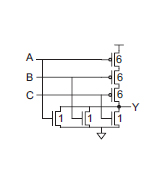##### Figure 3. 3-Input NOR gate

This approach can be used to calculate the logical efforts of other common logic gates as shown in the table below.

Table 1. Logical effort of common gates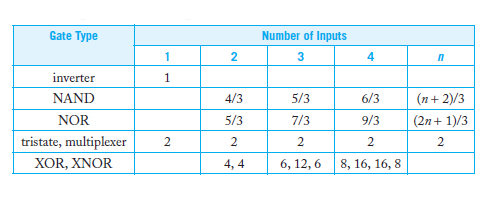Parasitic delay can be calculated using the Elmore delay as described in the previous article[link] or by simulation. To briefly summarize the effect of the parasitic delay, consider an N-input NAND gate and it Elmore delay equivalent value is given in the figure below;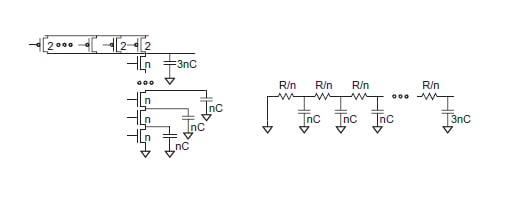##### Figure 4. N-input NAND gate and RC equivalent circuit

The Elmore delay is,

$t_{pd}=R(3nC)+ \sum_{i=1}^{n-1}(\frac{iR}{n})(nC)=\left ( \frac{n^2}{2}+\frac {5}{2}n \right )RC$

From the expression, the parasitic delay part is seen to increase quadratically with the number(n) of series transistors, which is why it is advisable to cascade two smaller gates to form a larger one to reduce the input parasitic delay of a gate. The implication of this can be further observed in a multistage logic network which is the sum of the delay of each stage in the path as shown in the multistage logic network shown in the figure below.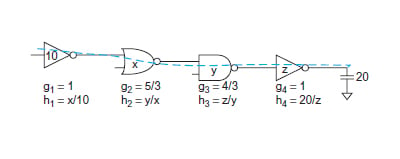##### Figure 5. Multistage logic network

$D=\sum{d_i} \; \;\textit{where} \; \; d_i= g_ih_i+p_i$

$D=\sum{g_ih_i} + \sum{p_i}$

$D=\sum{g_ih_i} + P \;\; \textit{where} \; g_ih_i = \textit{stage effort}$

$F=\prod {f_i}=\prod {g_ih_i}=GH \textit{(stage effort)}$

The path electrical effort, H,  is the ratio of the output capacitance of the path to the input capacitance of the path.

$H = \frac {\textit{Capacitance at the output}}{\textit{Capacitance at the input}}= \prod{h_i}$

The path effort F is the product of the stage effort of each stage.  Since the goal is to arrive at the minimum delay value each stage will contribute an equal amount of stage effort to an n-stage critical path. It is required that each stage must have equal stage effort. Therefore for an N-stage network, the minimum stage effort is simply;

$$f_i=F^{\frac{1}{N}}$$-----minimal stage effort

Therefore,

$\sum{g_ih_i}=Nf_i$

Therefore the delay expression can be written as

$d = NF^{\frac{1}{N}} +P$

To better understand how this concept is applied in a real-world system,  we are going to consider a functional unit required to drive 64 units of capacitance via an inverter. With the knowledge of the logical effort, we can determine the optimal number of stages to provide the minimum delay.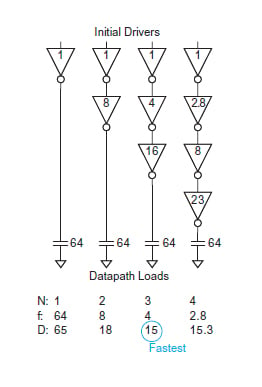##### Figure 6. Comparison of different number of stages of inverter buffers

Note that the parasitic delay $$p_i$$ for an inverter is 1, so for an N-stage inverter chain network, the total parasitic delay P of the path will be the sum of the parasitic delay of each inverter, which is N.

N = 1 N = 2 N = 3 N = 4
$\textit{Recall},d =NF^{\frac{1}{N}}+P$ $\textit{Recall},d =NF^{\frac{1}{N}}+P$ $\textit{Recall},d =NF^{\frac{1}{N}}+P$ $\textit{Recall},d =NF^{\frac{1}{N}}+P$
$P=\sum{p_i}=N =1$ $P=\sum{p_i}=N =2$ $P=\sum{p_i}=N =3$ $P=\sum{p_i}=N =4$
$F=GH = \frac{64}{1} =64$ $F=GH = \frac{64}{1} =64$ $F=GH = \frac{64}{1} =64$ $F=GH = \frac{64}{1} =64$
$f_i =64^{\frac{1}{1}}=64$ $f_i =64^{\frac{1}{2}}=8$ $f_i =64^{\frac{1}{3}}=4$ $f_i =64^{\frac{1}{4}}=2.83$

$d=(1)64^{\frac{1}{1}}+1$

$d=(2)64^{\frac{1}{2}}+2$ $d=(3)64^{\frac{1}{3}}+3$ $d=(4)64^{\frac{1}{4}}+4$
$d=65$ $d=18$ $d=15 (\textit{lowest})$ $d=15.3$

Since the 3-stage inverter design is the optimal design with the lowest delay, we shall determine the size of the inverter in each stage to give the optimal delay.

Since each stage effort $$f_i = 4$$, then the first inverter close to the load capacitance is sized as,

$FI= \frac{64}{C_{in}}, CIN=\frac{64}{4}=16 \;\textit{UNIT}$

The middle inverter is derived as $$FI= \frac{16}{C_{in}}, CIN=\frac{16}{4}=4 \;\textit{UNIT}$$

While the size of the first inverter at the input is derived as,

$FI= \frac{4}{C_{in}}, CIN=\frac{4}{4}=1 \;\textit{UNIT}$

The summary of each stage is shown in figure 6 above. As can be observed, a one-stage inverter network provides the largest delay, even-though the overall size of the circuit will be minimum, the design will run extremely slow. While the 3-stage design will have more area, it provides the best amount of delay to the design, hence making the system work according to specification.

So far, we have been able to investigate the various delay models in the MOS circuit. While these methods are simple and reasonably accurate, they should not serve as a complete replacement for CAD tools in situations where accuracy is crucial. However, these methods are suitable to provide a quick and dirty approach to develop insights into various circuit topologies and critical paths design.